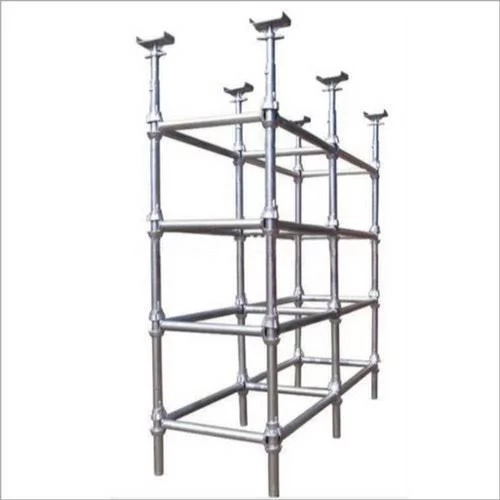# How To Calculate The SWL Of A ScaffoldKnowing how to calculate  the SWL of the scaffold you intend to work on is very important as it will prevent you from overloading the scaffold.

Before we get down to how to calculate the SWL of a scaffold, let’s define what SWL (Safe working load) is:

SWL is the maximum safe force that a piece of lifting equipment, lifting device or accessory can exert to lift, suspend, or lower a given mass without fear of breaking.

## How To Calculate The SWL Of A Scaffold

I came across this:

It can be calculated using this formula:

SWL = D + (4 x L)

Where –

– SWL = Safe Working Load

– D = Dead Load: This is the weight of the working platform and the scaffold components.

– L = Live Load: This include the weight of workers, material and tools

When all the variables in the formulae is obtained, fixing the values in, we can calculate the SWL of any scaffold.

Contributions are welcome

Related Articles

The concept of Safe Working Load (SWL)

Fire load of a building: Definition and how it is calculated